﻿ Parzen窗估计在潜艇目标强度统计建模的应用
 舰船科学技术2017, Vol. 39Issue (2): 132-136PDF
Parzen窗估计在潜艇目标强度统计建模的应用

1. 中国船舶重工集团公司第七〇五研究所, 陕西 西安 710075;
2. 水下信息与控制重点实验室, 陕西 西安 710075

Application of parzen window estimation in submarine target strength statistics modeling
SUN Nai-wei1,2, LI Jian-chen1, WAN Ya-min1
1. The 705 Research Institute of CSIC, Xi'an 710075, China;
2. Science and Technology on Underwater Information and Control Laboratory, Xi'an 710075, China
Abstract: The statistics characteristics research of submarine target strength (TS) have important value to the simulation and experiment analysis of underwater weapon. Aimed at the problem that histogram statistics model precision is quite affected by area partition, Parzen window estimation method is used for submarine TS statistics modeling in this paper. Submarine TS distribution model in typical bow bearing is built and the statistics characteristics is analyzed. Benchmark submarine is used for simulation, the simulation results show that submarine TS statistics model by Parzen window estimation measures up to submarine physical structure property and it can forecast submarine TS statistics characteristics more accurately and more effectively.
Key words: target strength     statistics characteristics     histogram     parzen window estimation

1 理论基础 1.1 目标强度板块元计算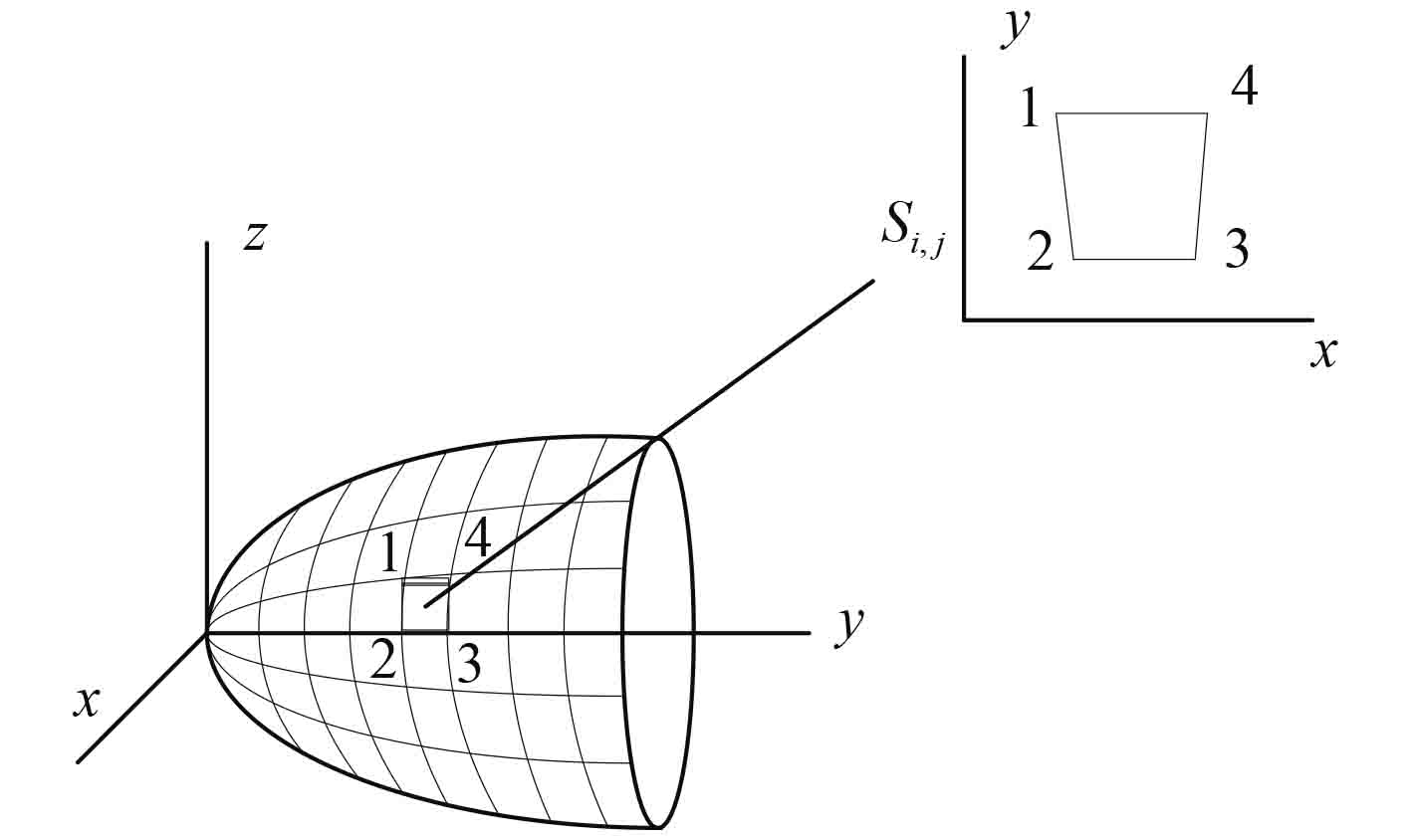图 1 网格划分及坐标转换 Fig. 1 Meshing and coordinates conversion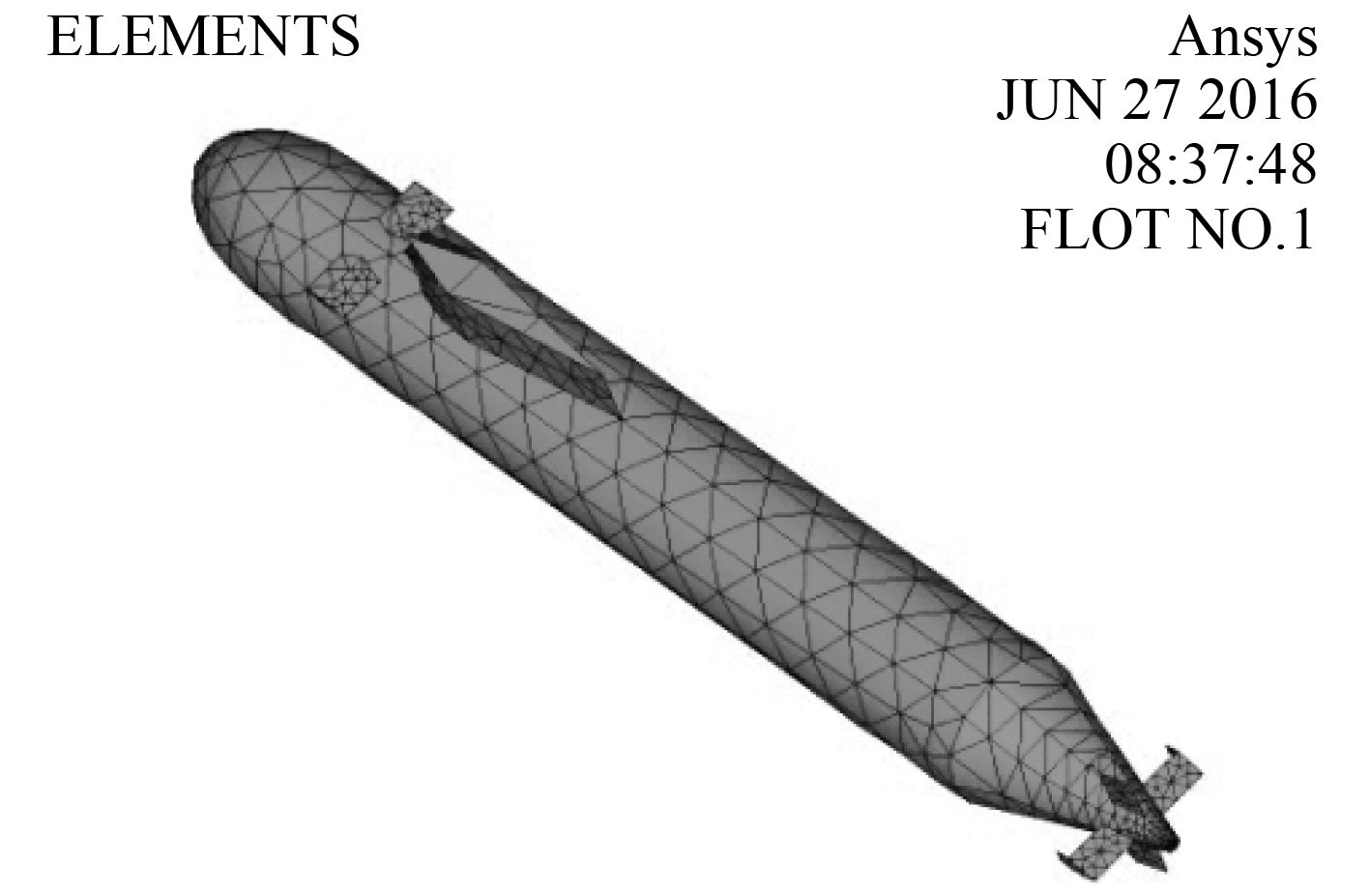图 2 Ansys 潜艇模型网格划分 Fig. 2 Submarine model meshing in Ansys
1.2 直方图统计建模

1） 将样本数据值覆盖的数据区间C 分成几个等子区间。

2） 对样本集D 内的N 个样本数据进行从小到大的排序，按照顺序依次判断样本数据值落到这个相应的子区间，这个子区间的高度就相应的加一个单位的高度。

3） 将各个子区间的高度进行归一化，建立直方图的概率统计模型。

1.3 Parzen 窗估计原理

 $P=\int {p\left( x \right){\rm d}x} \text{，}$ (1)

 $Bin\left( {K|N,P} \right)=\frac{{N!}}{{K{\rm{!}}\left( {N - K} \right)!}}{P^K}{\left( {1 - P} \right)^{1 - K}}\text{，}$ (2)

 ${\mathop{\rm p}\nolimits} \left( x \right)=\frac{K}{{NV}}\text{。}$ (3)

1） K 不变，通过决定区域V 的大小来估算密度函数。

2） V 不变，通过决定K 的大小来估算密度函数。

 $p\left( x \right)=\frac{1}{N}\sum\limits_{n=1}^N {\frac{1}{{{h^D}}}k\left( {\frac{{x - {x_n}}}{h}} \right)} \text{，}$ (4)

 $k\left( u \right)=\frac{{\rm{1}}}{{\sqrt {2 } }}{\rm{exp[ - }}\frac{1}{2}{{\mathop{u}\nolimits} ^2}] \text{。}$ (5)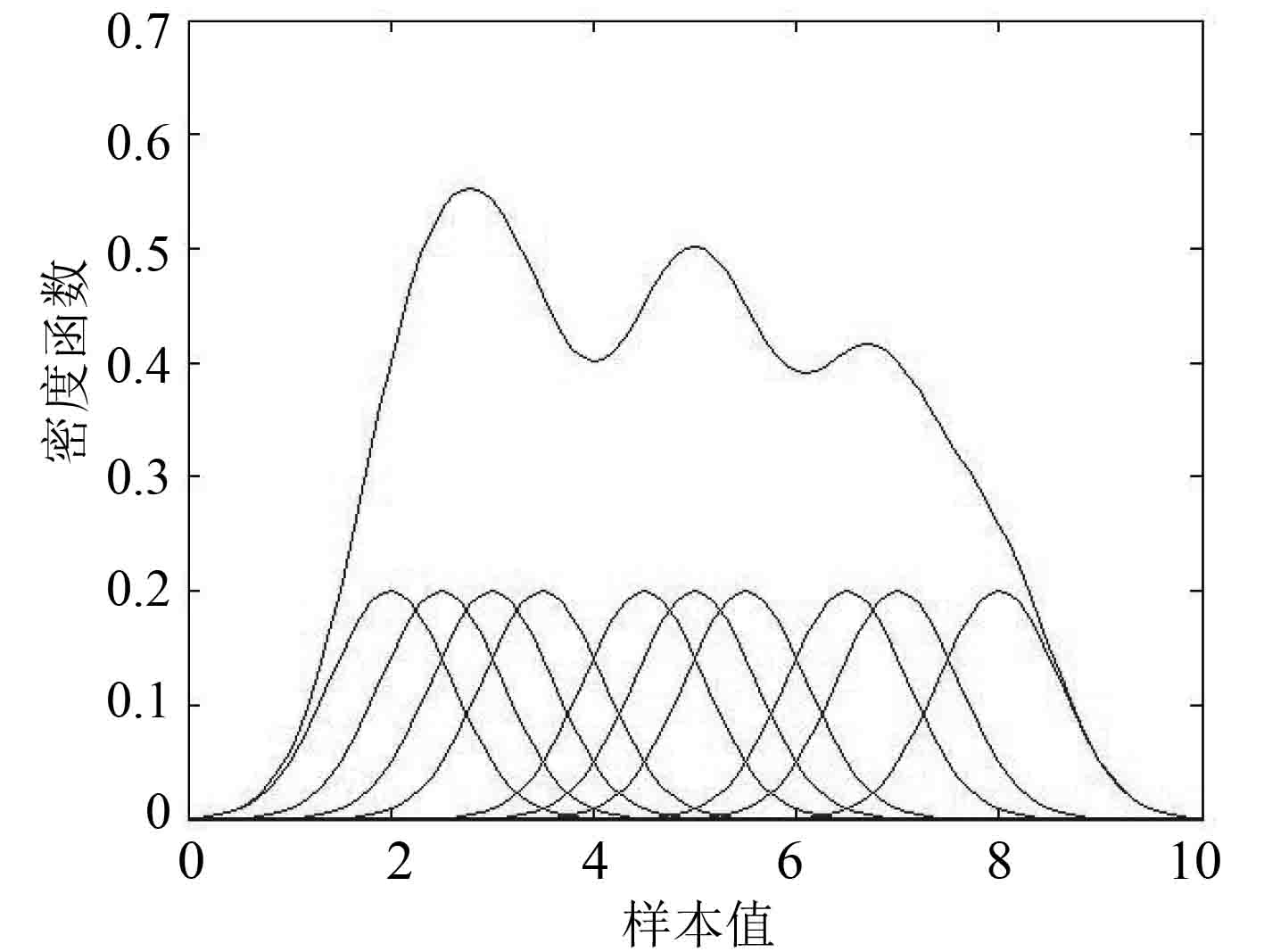图 3 Parzen 窗估计法原理图 Fig. 3 Schematic diagram of Parzen window estimation method
2 建模与仿真计算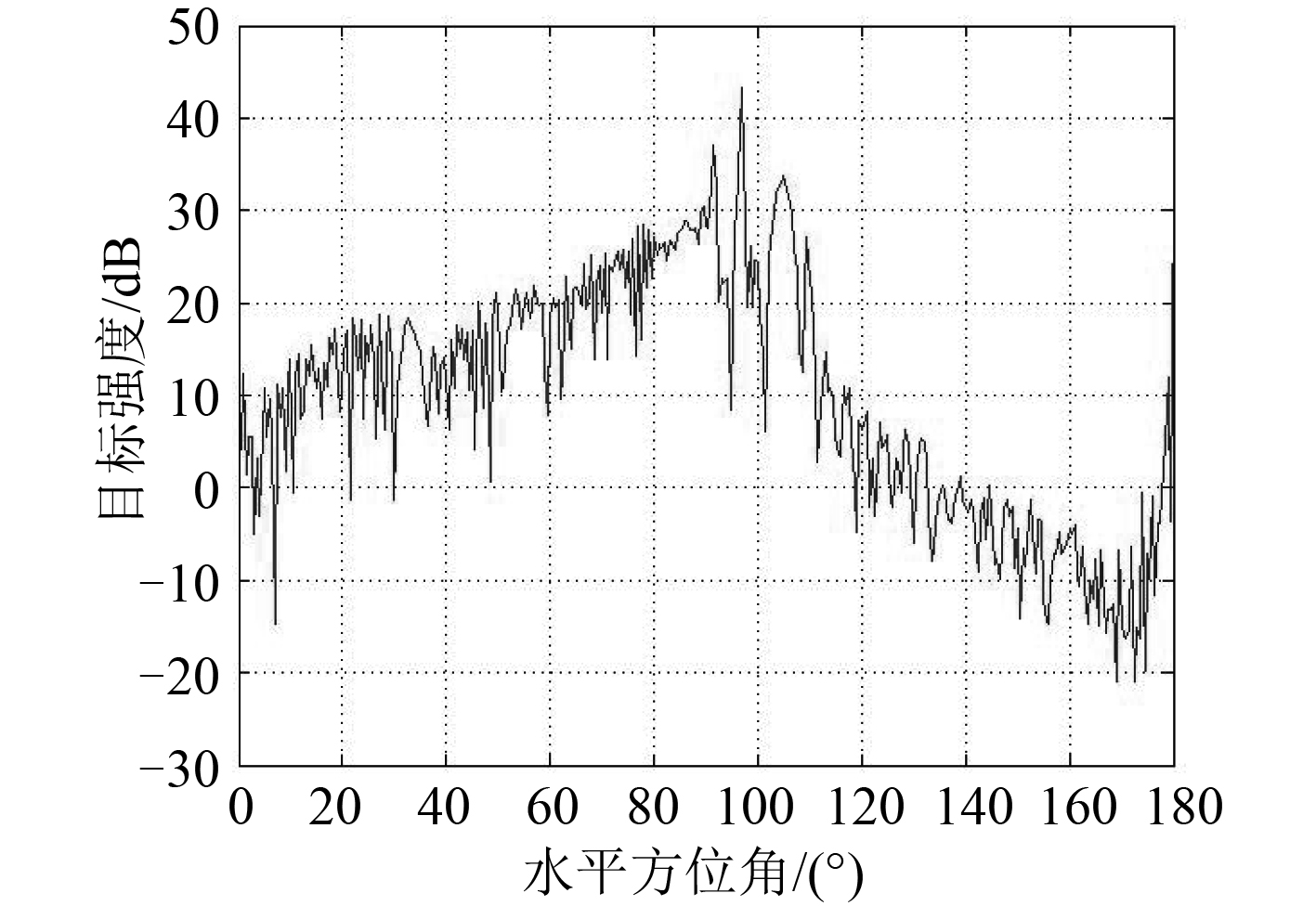图 4 潜艇目标强度曲线 Fig. 4 Submarine target strength graph

2.1 典型舷角下的直方图统计建模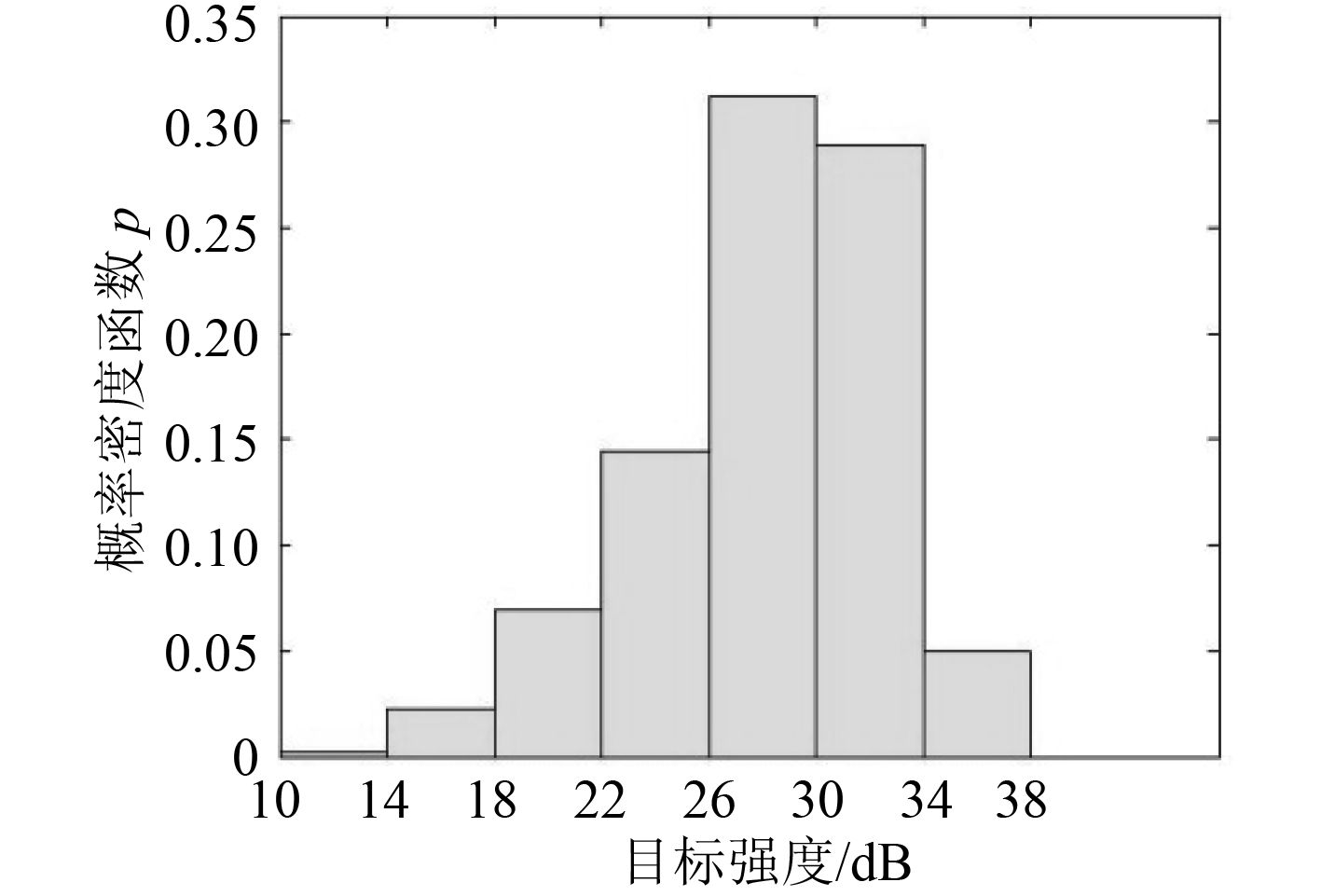图 5 d=4 时中部目标强度分布 Fig. 5 TS distribution in midship whend=4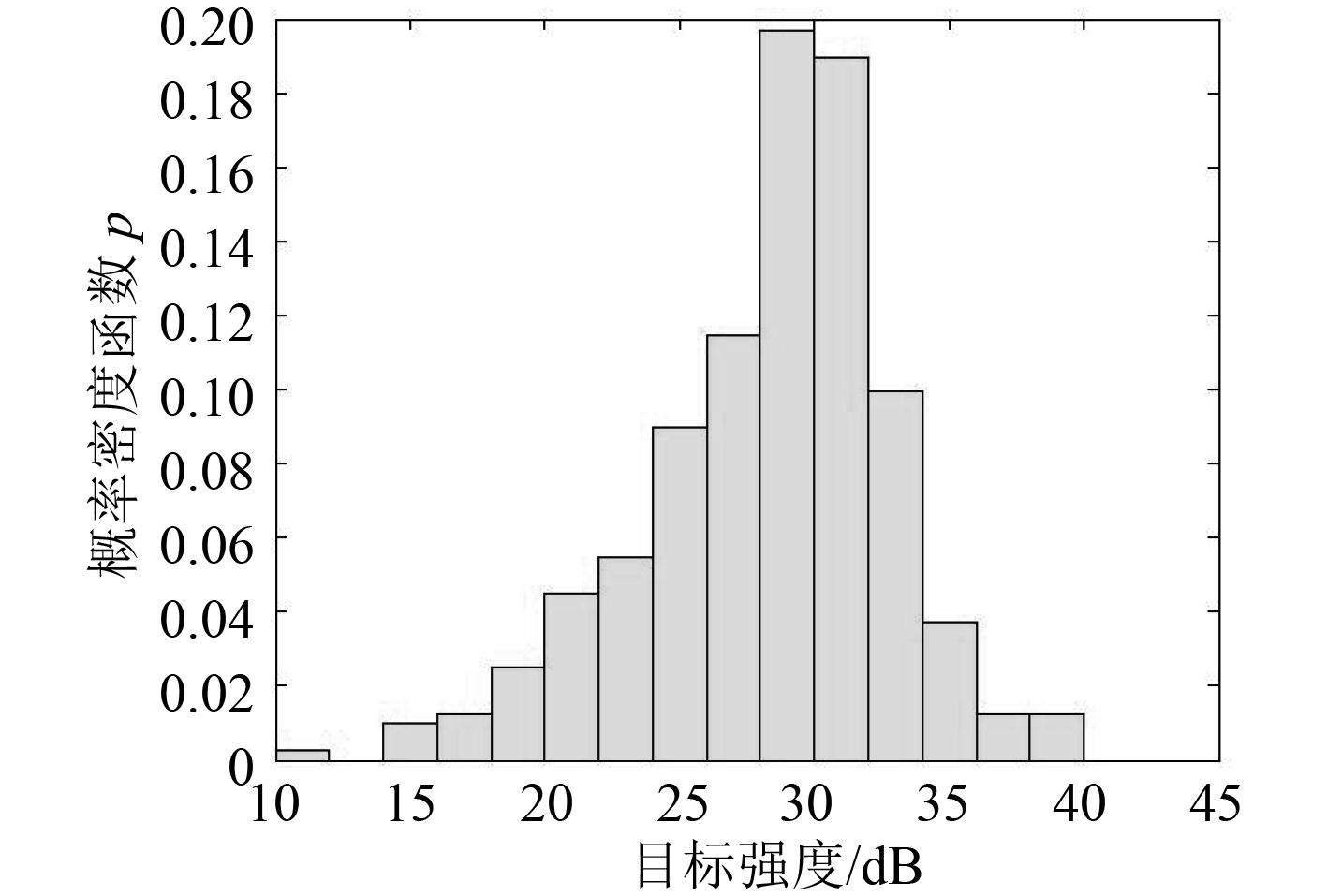图 6 d=2 时中部目标强度分布 Fig. 6 TS distribution in midship whend=2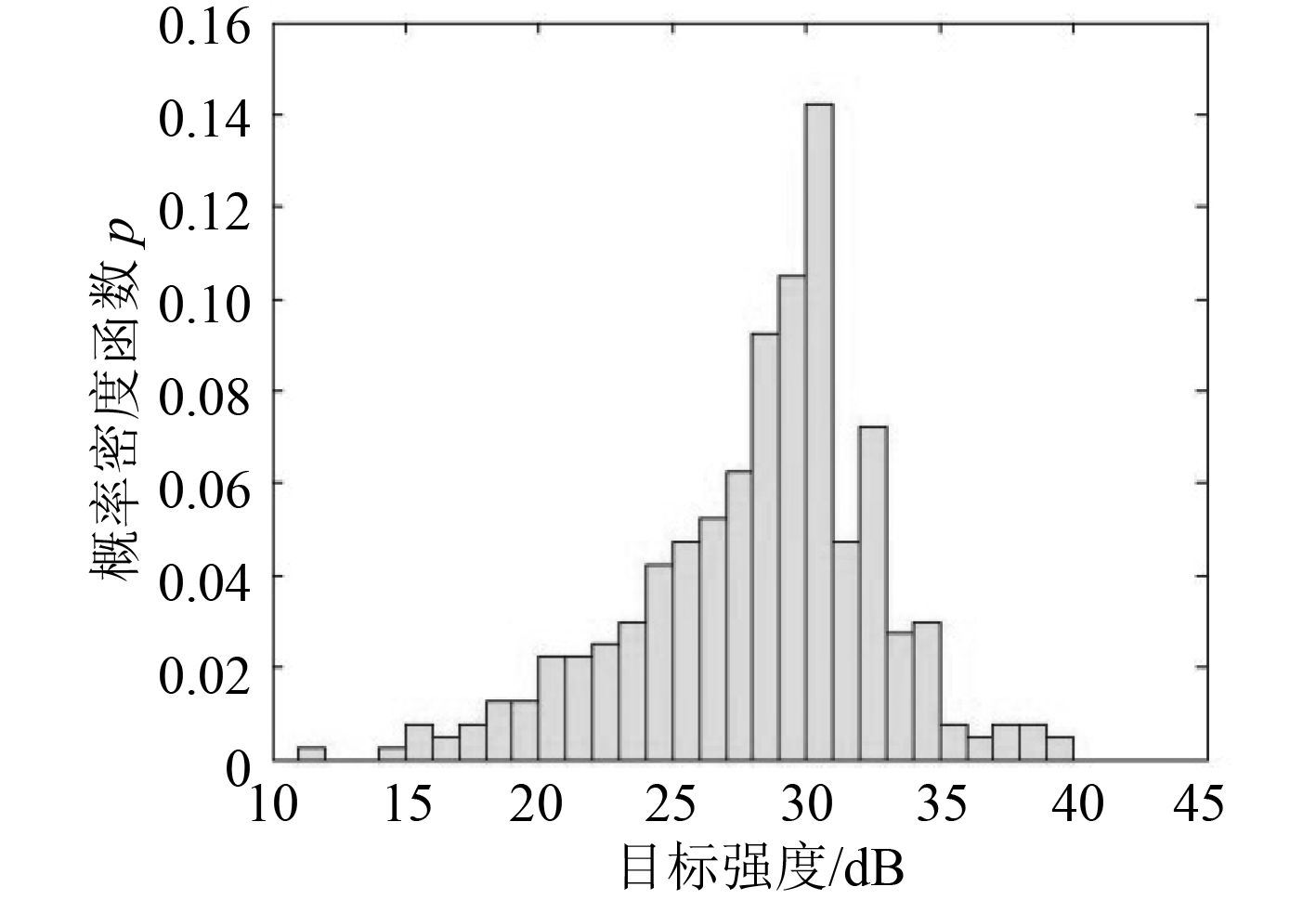图 7 d=1 时中部目标强度分布 Fig. 7 TS distribution in midship whend=1

2.2 Parzen窗估计法有效性验证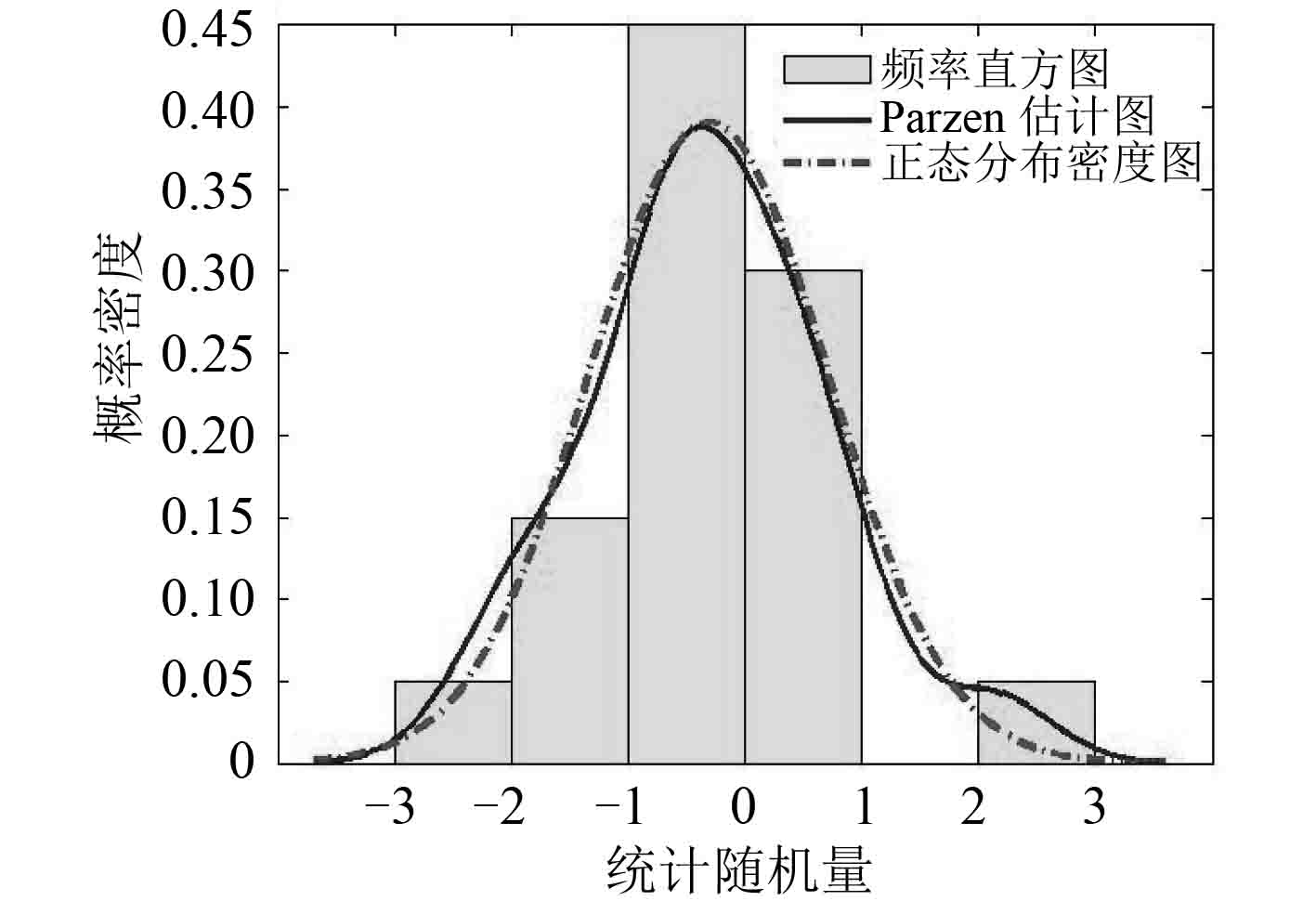图 8 样本数N=20 Fig. 8 Sample numberN=20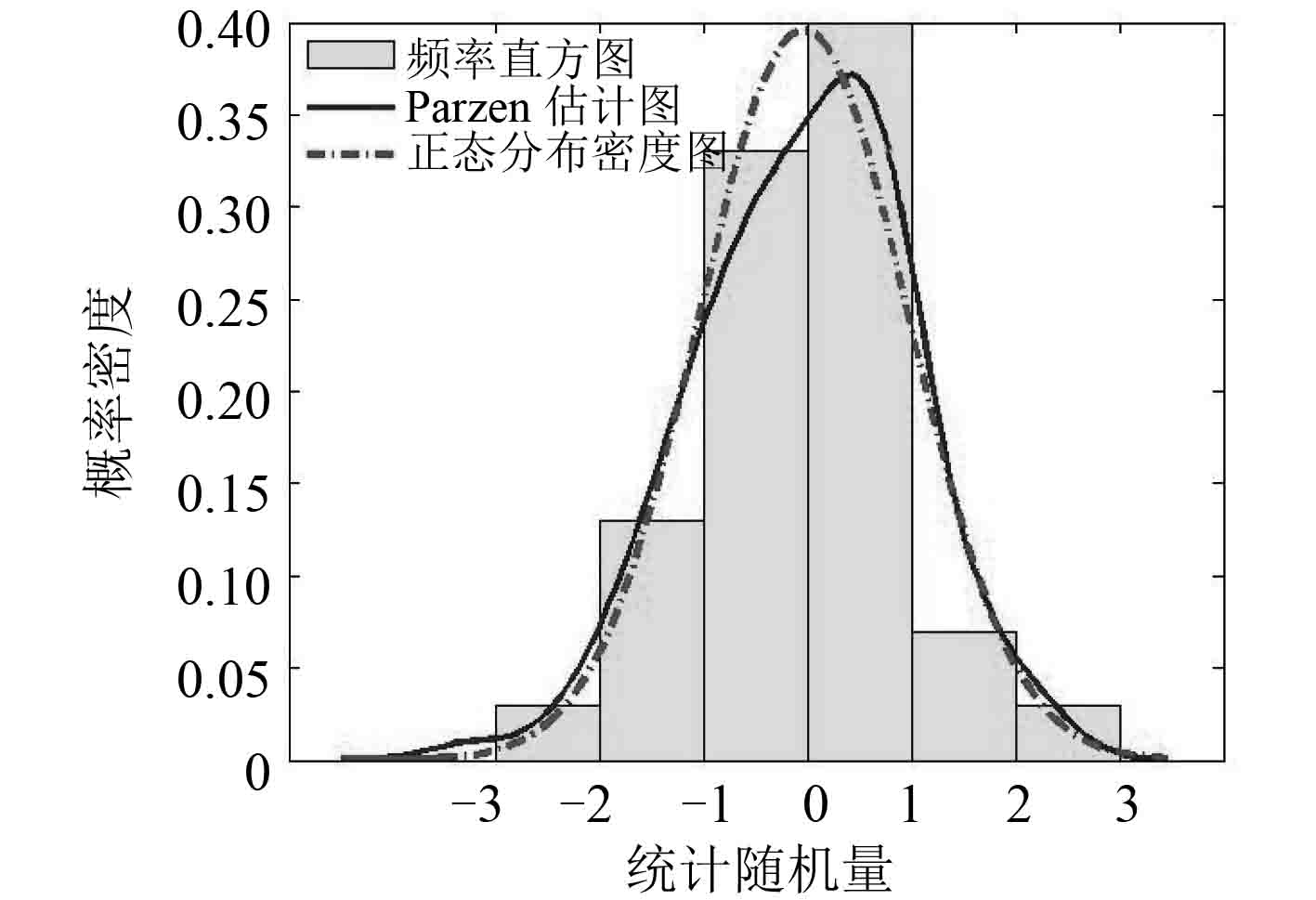图 9 样本数N=100 Fig. 9 Sample numberN=100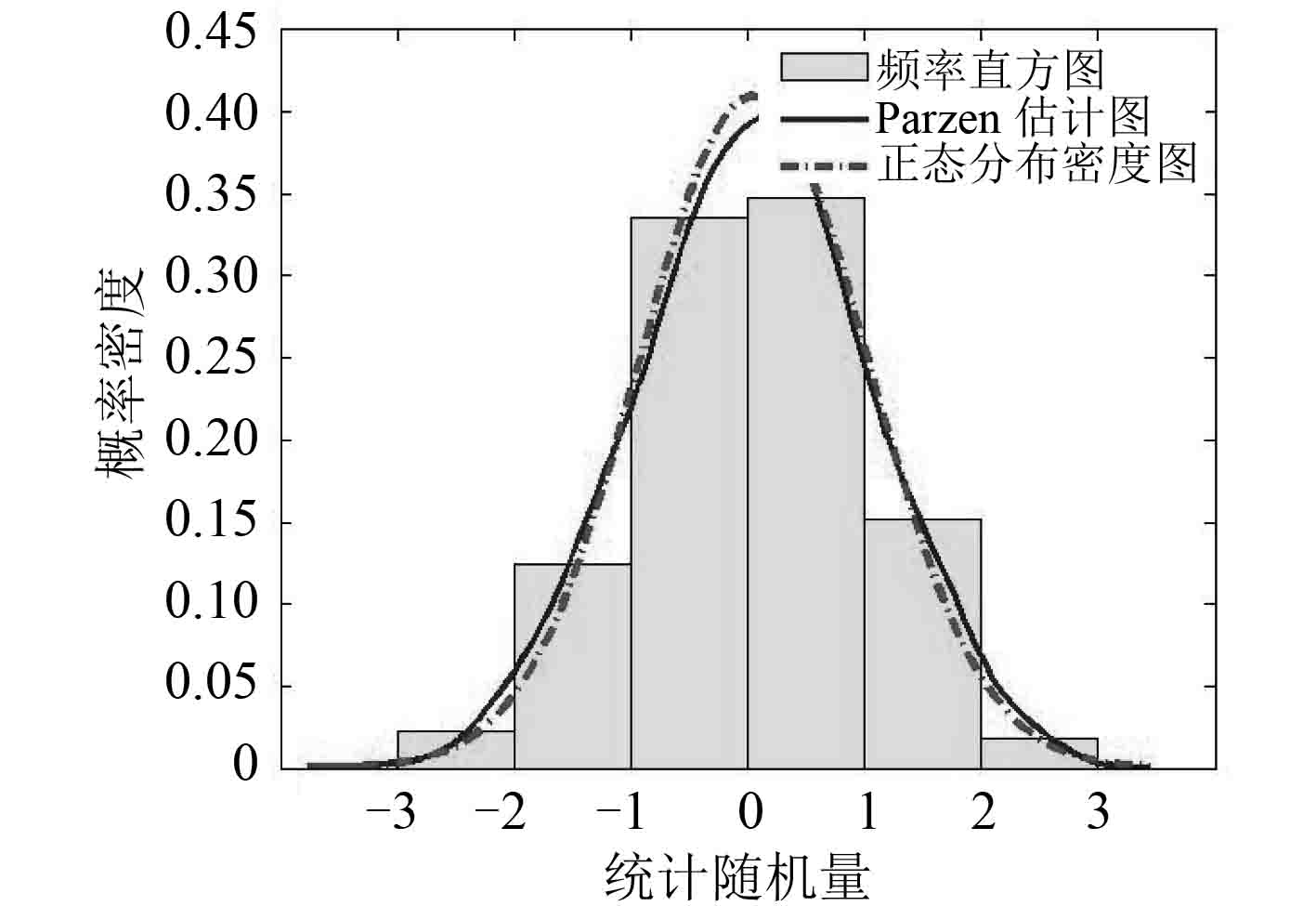图 10 样本数N=500 Fig. 10 Sample numberN=500

2.3 Parzen 窗估计法建模与计算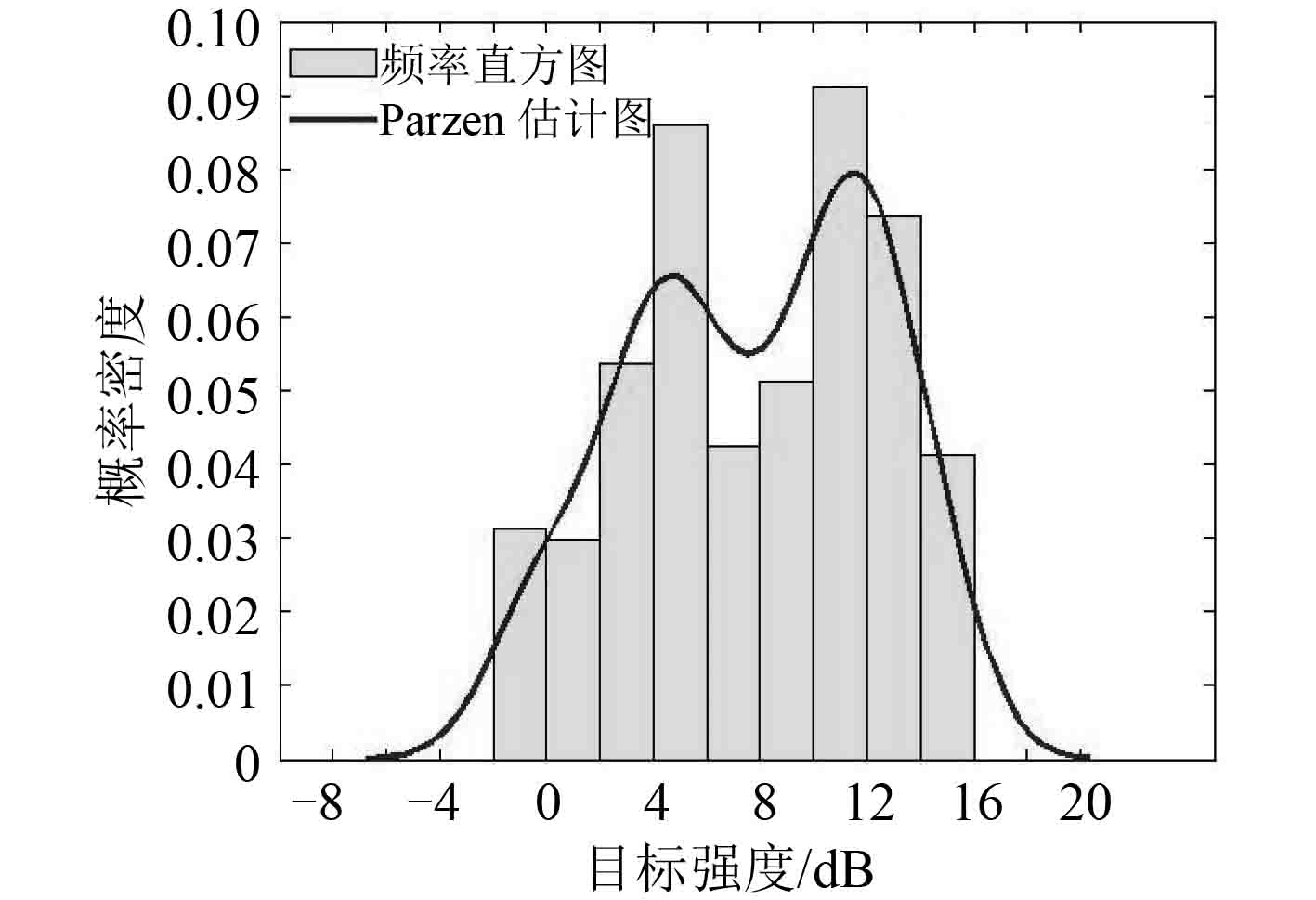图 11 首部目标强度分布 Fig. 11 TS distribution in bow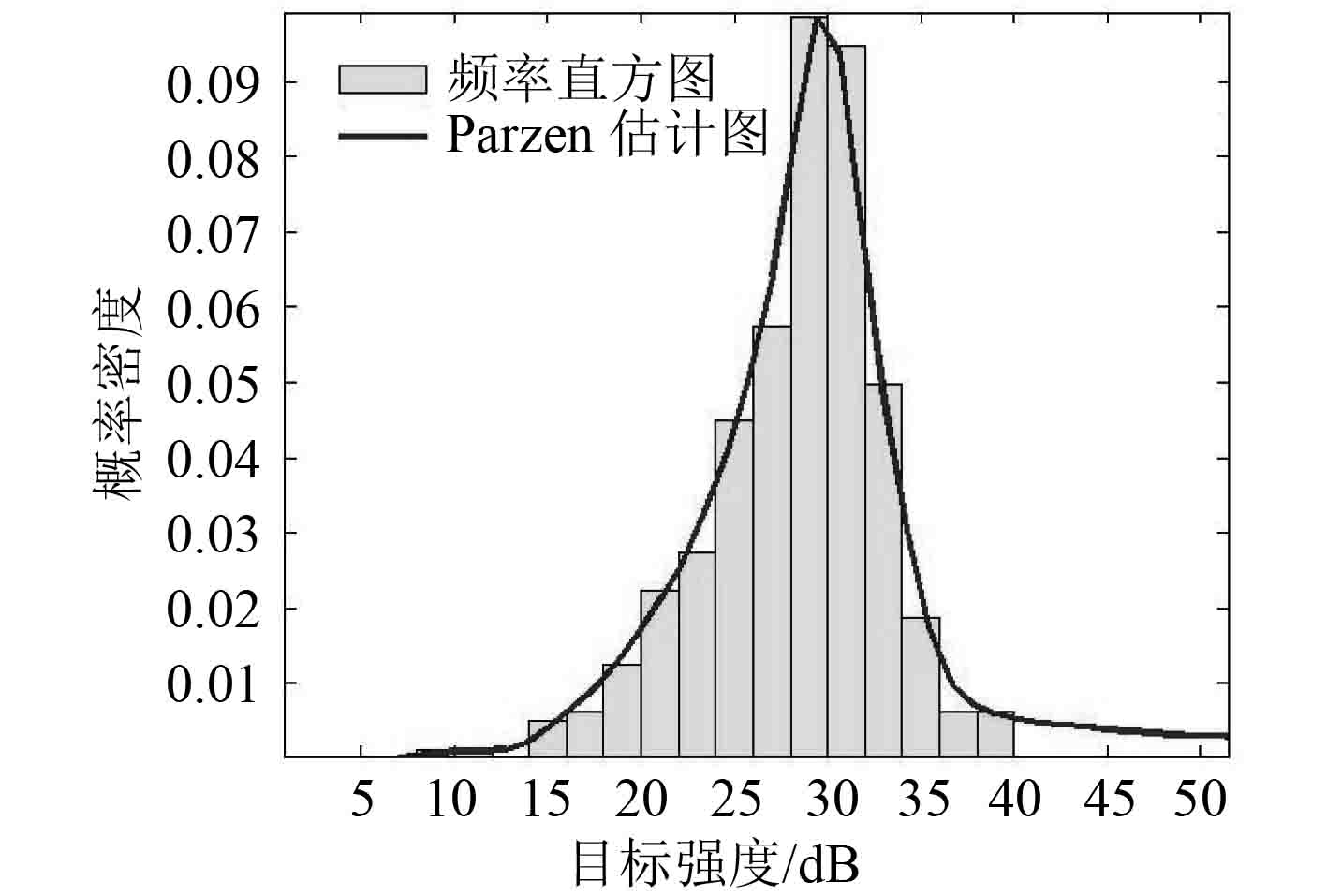图 12 中部目标强度分布 Fig. 12 TS distribution in midship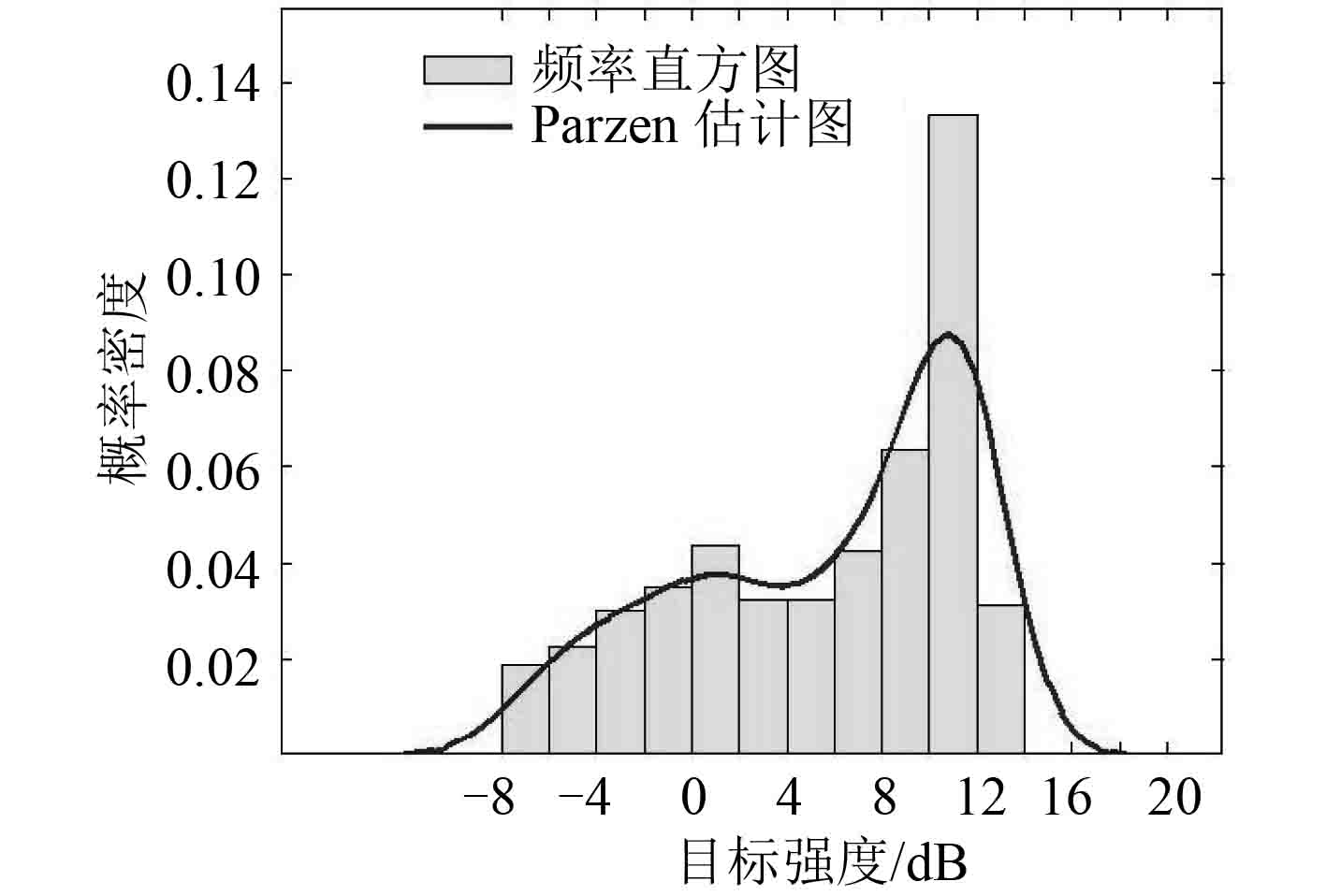图 13 尾部目标强度分布 Fig. 13 TS distribution in stern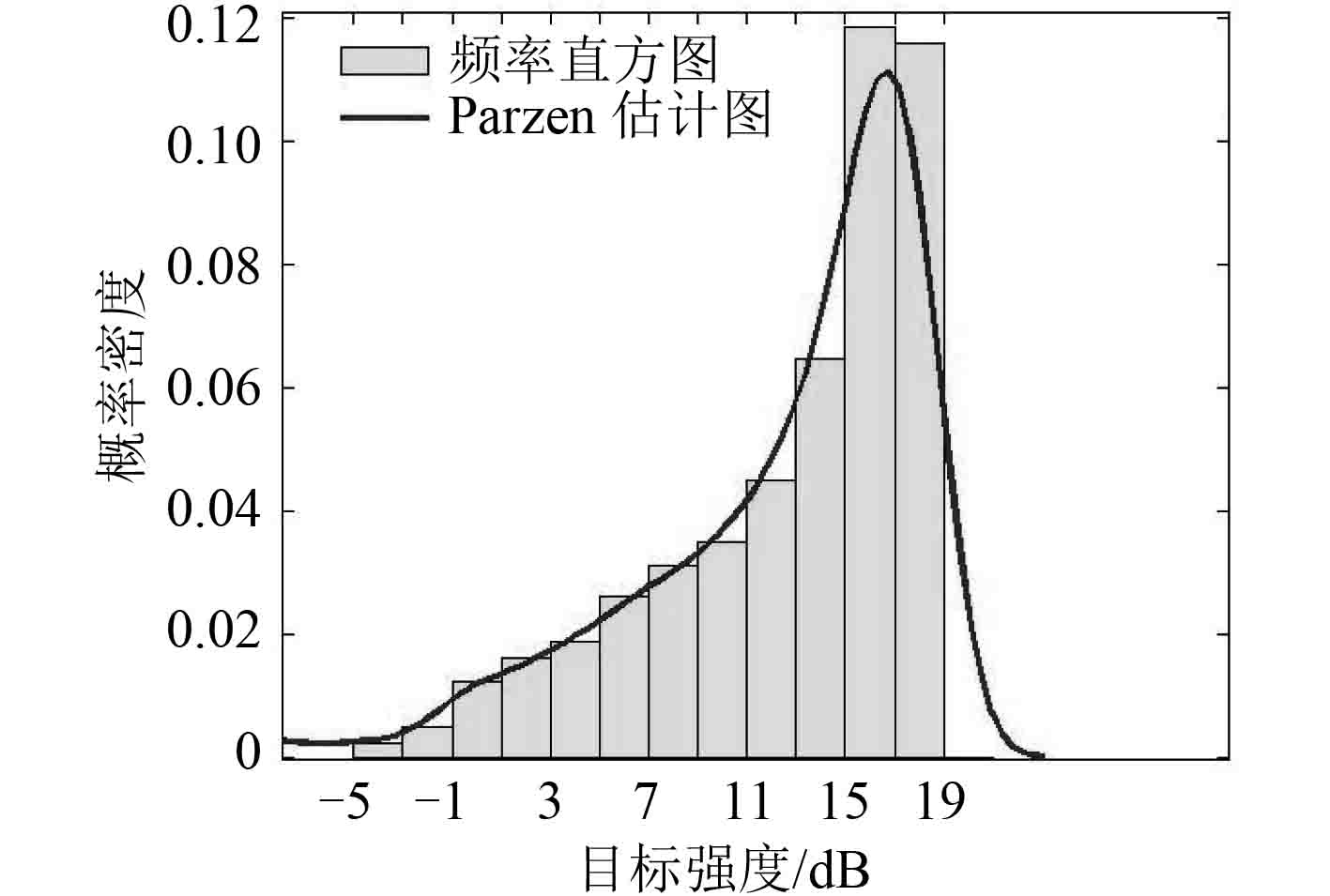图 14 30° 舷角目标强度分布 Fig. 14 TS distribution in bow bearing of 30°

1） 潜艇中部的平均目标强度最大，其次是 30° 舷角处的平均目标强度，均高于艇首和艇尾的平均目标强度，各个典型舷角下的目标强度分布具有连续性，仿真结果符合潜艇目标强度经典的蝶形图分布规律。

2） 潜艇在各个舷角的目标强度值均具有明显的起伏且在不同入射角度下的目标强度的密度分布特征各不一样，其中潜艇中部的目标强度分布较集中，首部和尾部目标强度分布范围较分散，符合目标曲率半径大小对目标强度变化的影响规律。

3） 采用 Parzen 窗估计法建立的潜艇目标强度分布模型较平滑和连续，便于提取统计参数，可直观有效地用于潜艇目标强度的统计分析。

3 结 语

  何心怡, 张鹏, 王光宇, 等. 大俯仰角时的潜艇目标强度研究[J]. 舰船科学技术, 2007, 29 (3): 37–39. HE Xin-yi, ZHANG Peng, WANG Guang-yu, et al. Target strength research of submarine under large pitching angle[J]. Ship Science and Technology, 2007, 29 (3): 37–39.  张杨梅, 冯西安. 气泡群目标强度的起伏统计模型[J]. 计算机仿真, 2005, 32 (9): 14–18. ZHANG Yang-mei, FENG Xi'an. Statistical model of target strength model of bubble cloud[J]. Computer Simulation, 2005, 32 (9): 14–18.  李建龙. 水下目标的目标强度统计模型研究[D]. 上海:上海交通大学, 2013. LI Jian-long. Research on the statistical model of target strength fluctuation of target strength fluctuation of underwater targets[D]. Shanghai:Shanghai Jiaotong University, 2013.  王汝夯, 李冰, 魏强. 基于板块元的水面舰船远场声目标强度预报方法[J]. 声学技术, 2015, 34 (1): 6–10. WANG Ru-hang, LI Bing, WEI Qiang. A method for predicting far-field target strength of surface ship based on planar elements theory[J]. Technical Acoustics, 2015, 34 (1): 6–10.  赵瑶, 常发亮. 直方图统计方法的对比研究[J]. 计算机技术与自动化, 2007, 26 (4): 71–74. ZHAO Yao, CHANG Fa-liang. The comparative study on histogram computation methods[J]. Computing Technology and Automation, 2007, 26 (4): 71–74.  陈银超, 杨伟. 基于Parz窗估计的电液伺服作动器故障检测与健康评估[J]. 计算机测量与控制, 2012, 20 (8): 2083–2088. CHEN Yin-chao, YANG Wei. Electric hydraulic servo actuator fault detection and health assessment based on parzen window estimation[J]. Computer Measurement & Control, 2012, 20 (8): 2083–2088.  张文成, 周穗华, 陈聪聪, 等. 板块元法求目标散射特性中面元划分方法研究[J]. 武汉理工大学学报(交通科学与工程版), 2014, 38 (4): 878–882. ZHANG Wen-cheng, ZHOU Hui-hua, CHEN Cong-cong, et al. Research on element partition of planar element method in target scattering characteristic calculation[J]. Journal of Wuhan University of Technology (Transportation Science & Engineering), 2014, 38 (4): 878–882.  汤阿妮. 基于核密度估计算法的飞机载荷谱统计技术[J]. 北京航空航天大学学报, 2011, 37 (6): 654–657. TANG A-ni. Technique of aircraft loads spectrum statistics based on kernel density estimation[J]. Journal of Beijing University of Aeronautics and Astronautics, 2011, 37 (6): 654–657.